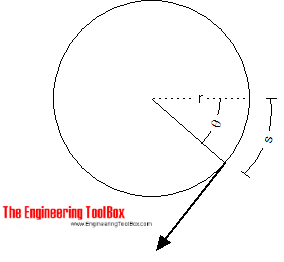Engineering ToolBox - Resources, Tools and Basic Information for Engineering and Design of Technical Applications!

# Torque - Work done and Power Transmitted

## The work done and power transmitted by a constant torque.

### Work done

Work done is the force multiplied with the distance moved by the force - and can be expressed as

W = F s                                  (1)

where

W = work done (J, Nm)

F = force (N)

s = distance moved by force (s)

For an angular motionthe work done can be expressed as

W = F θ r

= T θ                                     (2)

where

W = work (Joules)

T = torque or moment (Nm)

### Power transmitted

Power is the ratio between the work done and the time taken and can be expressed as

P = W / dt

= T θ / dt

= T ω

= 2 π n T

= 2 π (nrpm / 60) T

= 0.105 nrpm T                                  (3)

where

P = power (Watts)

dt = time taken (s)

ω =  θ / dt = 2 π n = angular velocity (rad/s)

n = speed (rev/s)

nrpm = speed (rev/min, rpm)

Note! - a machine must rotate to produce power! A machine with no rotation can deliver torque - like an electric motor - but since no distance is moved by force - no power is produced. As soon as the machine starts to rotate power is produced.

### Example - required Torque to produce Power

A machine rotates with speed 3000 rev/min (rpm) and consumes 5 kW. The torque at the shaft can be calculated by modifying (3) to

T = P / 2 π n

= (5 kW) (1000 W/kW) / 2 π (3000 rev/min) / (60 sec/min)

= 15.9 Nm

## Related Topics

• Mechanics - Forces, acceleration, displacement, vectors, motion, momentum, energy of objects and more.

## Engineering ToolBox - SketchUp Extension - Online 3D modeling!

Add standard and customized parametric components - like flange beams, lumbers, piping, stairs and more - to your Sketchup model with the Engineering ToolBox - SketchUp Extension - enabled for use with the amazing, fun and free SketchUp Make and SketchUp Pro .Add the Engineering ToolBox extension to your SketchUp from the SketchUp Pro Sketchup Extension Warehouse!

Translate

## Privacy

We don't collect information from our users. Only emails and answers are saved in our archive. Cookies are only used in the browser to improve user experience.

Some of our calculators and applications let you save application data to your local computer. These applications will - due to browser restrictions - send data between your browser and our server. We don't save this data.

## Citation

• Engineering ToolBox, (2008). Torque - Work done and Power Transmitted. [online] Available at: https://www.engineeringtoolbox.com/work-torque-d_1377.html [Accessed Day Mo. Year].

Modify access date.

. .

#### Scientific Online Calculator3 30